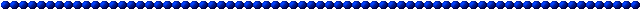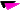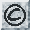# Task-Arrays

Sets of tasks spanning problem dimensions.When comparing learning methods, we are interested in more than just a single overall performance measure. We expect each learning method to have particular strengths and weaknesses that are revealed by particular types of task. Some may model complicated non-linearities well but overfit badly on noisy data. Others may work well for low-dimensional data but suffer badly from the curse of dimensionality. We would like to compare learning methods along many different dimensions, but practical considerations force us to restrict our attention to just a few of the most important characteristics of a task.

Using natural tasks it is hard to generate a balanced design in which different characteristics of the task are varied systematically. With simulated or artificial tasks this is far easier to achieve. Delve includes a number of task-arrays that have been specifically designed to make it easy to compare regression methods along the following dimensions:Dimensionality of the input (8 or 32)Amount of noise in the output (moderate or high)Degree of non-linearity of the underlying function (fairly linear or non-linear)

When combined with five different sizes of training set (64, 128, 256, 512, 1024), these three binary dimensions give rise to task-arrays that each contain 40 tasks. For each of these tasks (except those with 1024 training cases) there are 8 different task-instances so that the effects of the sampling noise in the training set can be estimated. For tasks with 1024 training cases there are only 4 task-instances. A learning method therefore has to be run on 288 task-instances for each task-array.

By using several such arrays we can gain confidence that the comparative performances are not an idiosynchratic property of a specific type of task. Although this design is far from perfect, it should give a much better characterization of a learning method than just picking a few tasks that differ from one another in uncontrolled ways.

For simulated tasks, it is usually easy to control the dimensionality, the noise-level and the degree of non-linearity. Dimensionality can be reduced by just freezing the values of some of the input variables. Noise level can be increased by adding some form of noise to the output variables or by unfreezing some input variables that are not included in the input vectors for the task. If the mapping from inputs to outputs is continuous, the degree of non-linearity can generally be reduced by restricting the ranges of the input variables. This is especially true for simulations of continuous physical processes which are usually locally linear.

We define the amount of noise in the output as the fraction of the variance that would remain unexplained if we used a universal approximator on an infinite training set. If this residual variance is less than 0.25% the noise is "low". If it lies between 1% and 5% the noise is "moderate". If it exceeds 25% the noise is "high". It is easy to estimate the noise level for simulated or artificial tasks that are produced by using a deterministic mapping from inputs to outputs and then adding noise. It is also easy to measure the noise level when the mapping is stochastic. For natural tasks or simulated tasks that involve the inversion of a generative model, it is much harder to estimate the noise level. Suppose, for example, that we use a deterministic generative model to map from a space of latent variables to a space of observable variables and the task is to predict one or more of the latent variables from the observables. It is possible that many different settings of the latent variables could give rise to the same observables, so there is some irreducible squared error. In such cases, we can use a number of non-linear methods on a very large training set to get an upper bound on the irreducible squared error. We can then add noise to the outputs to ensure a lower bound. This introduces some bias in the selection of tasks, but this bias is mitigated by the fact that we only test the non-linear methods on much smaller training sets.

The degree of non-linearity is easy to define when there is no noise in the outputs. A task is "fairly linear" if a linear method would leave less than 5% residual variance on an infinite training set. It is "highly non-linear" if the linear method would leave more than 40% residual variance. Since linear methods are fast to train and relatively well defined for large training sets it is easy to measure the residual variance. When there is noise in the outputs, we define the degree of non-linearity in terms of the part of the variance that is not noise. If, for example. a linear model with an infinite training set leaves less than 5% of this variance unexplained the task is fairly linear. This definition has the advantage that adding noise to the outputs does not change the degree of nonlinearity, but it also has disadvantages. When there is a lot of noise, non-linear methods may only do slightly better than linear methods on highly non-linear tasks with large training sets. Also, to determine the degree of non-linearity it is necessary to know how much of the variance to attribute to noise and we can sometimes only get an upper bound on this noise level.

 Last Updated 16 September 1996 Comments and questions to: delve@cs.toronto.edu